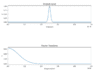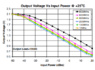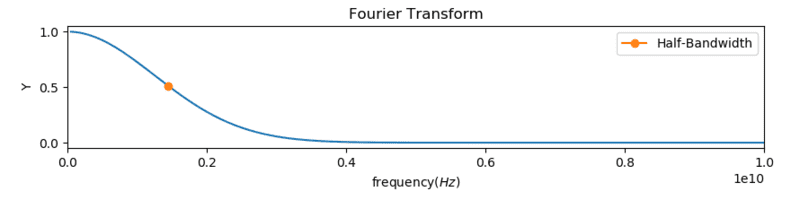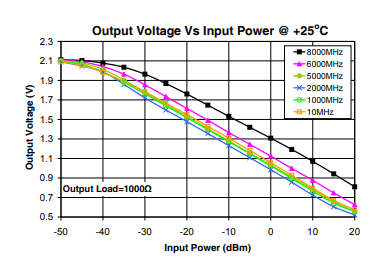# RF pulse detection with Power Detectors

#### Jiho

How can I use power detectors for RF pulse detection? RF pulse has a symmetric Gaussian-like shape.
Is the output of power detectors continuous line or point?

Last edited by a moderator:

#### berkeman

Mentor
Welcome to the PF.There are a number of different power detector types. Which type are you asking about? What is the repetition rate of the pulses (the duty cycle)? What power levels are involved? What RF frequencies are involved?

And is this really homework? If not, I can move your thread to the technical forums.

Last edited:

#### Jiho

I'm studying how to catch pulse width without oscilloscope. Unknown pulse is from Q-switched laser which has a symmetric Gaussian-like shape.
If a pulse is sinusoidal wave form, the problem becomes quite simple. I can find exact frequency because Fourier transform of sinusoidal wave form has finite number of frequency.But Fourier transform of Gaussian is Gaussian which has infinite number of frequency. That means each wave of frequency has different power. Is this right?This is the graph of power detector what I consider.I can't come up with which value is output from power detector. I am confused that the output of power detector is just one value or a continuous liner value.

Unfortunately, I don't know the type of power detector. Also I will use just one pulse so the duty cycle is not taken into consideration.

And.. if possible would you kindly explain me relation between RF pulse detection and power detector or advise me a web site?

P.S)When I posted this thread, I didn't knew about specific categories. I should have posted on the technical forum.

#### Attachments

• 32.4 KB Views: 64
• 41.6 KB Views: 70
• 32.3 KB Views: 73
• 4.7 KB Views: 144
• 1.8 KB Views: 147
• 8.4 KB Views: 147

#### berkeman

Mentor
If you want to detect the pulse width, you would use an envelope detector, not a power detector. Let me know the frequencies of interest and I can suggest an instrument.
P.S)When I posted this thread, I didn't knew about specific categories. I should have posted on the technical forum.
(Thread moved from the schoolwork forums to the technical forums)

#### tech99

Gold Member
How can I use power detectors for RF pulse detection? RF pulse has a symmetric Gaussian-like shape.
Is the output of power detectors continuous line or point?
If you use a power detector, the output voltage is proportional to input power irrespective of wave shape.
A typical power detector for lower frequencies is a square law device, like a diode. At optical frequencies a photo diode performs the same function. If you apply a sine voltage wave, the output is its square. If we average this over a period of time we obtain the mean square of the input voltage, and so it represents the mean square value of the input voltage waveform, which is the power.

If you have a pulsed waveform, the average power can be found by measuring the output voltage with an averaging circuit, such as a resistor followed by a shunt capacitor, giving a long time constant. Then you measure the peak power, now using a small resistor with the capacitor, so that RF cycles are smoothed but the envelope fully charges the capacitor, so now we see the peak power in the envelope.
Using average and peak power you can find the mark/space ratio, or more accurately, duty cycle, as we do not know the pulse shape.

#### Jiho

I have a Gaussian like shaped pulse waveform. It has 0.3ns pulse width, and it means 1.45Ghz half-band width in my calculation. If signal has 13dBm then what is output voltage from power detector? Is this enough information to calculate output or not? It's ok if I could get approximate value.#### Attachments

• 40.1 KB Views: 28
• 22.1 KB Views: 118
• 40.5 KB Views: 120

#### tech99

Gold Member
I have a Gaussian like shaped pulse waveform. It has 0.3ns pulse width, and it means 1.45Ghz half-band width in my calculation. If signal has 13dBm then what is output voltage from power detector? Is this enough information to calculate output or not? It's ok if I could get approximate value.
View attachment 237287
View attachment 237288
I don't like the fact that you have a DC offset on the power meter calibration. Best to re-draw the graph with 2.1 volts as the zero and reading upwards.
If 13dBm is the average power, then a power meter with an averaging CR circuit (or just a slow analogue meter) would read around 0.9 volt depending on carrier frequency.
If 13dBm is the peak power, then a power meter fitted with a peak reading capacitor will similarly read 0.9V. In this case I don't know what average reading you will get.

•Jiho

### The Physics Forums Way

We Value Quality
• Topics based on mainstream science
• Proper English grammar and spelling
We Value Civility
• Positive and compassionate attitudes
• Patience while debating
We Value Productivity
• Disciplined to remain on-topic
• Recognition of own weaknesses
• Solo and co-op problem solving